Next: Corollary 1.1 Up: Proof that Algorithm Increases Previous: Proof that Algorithm Increases   Contents

### Theorem 1

Theorem 1: Given the state vector of our register with one state with amplitude k, and every other state with amplitude l, after an application of A:

• the amplitude in the one state is k' =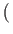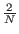- 1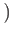k + 2l
• the amplitude of the remaining (N - 1) states is l' =k +l

Proof: Given the definition of A as Aij = 2/N if i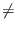j and Aii = - 1 + 2/N, it follows from the definition of matrix multiplication of k and l by A that:

• k' =- 1k + 2l
• l' =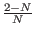l +k +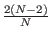l
The second expression simplifies to l' =k +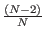l with some simple algebraic manipulation. [Grover96]Next: Corollary 1.1 Up: Proof that Algorithm Increases Previous: Proof that Algorithm Increases   Contents
Matthew Hayward - Quantum Computing and Grover's Algorithm GitHub Repository